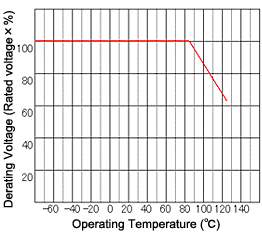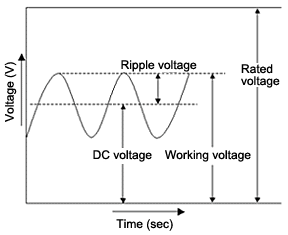Part Number Search

FAQ Category

FAQ's
showing 1 to 10 of 16
• TCs are electrolytic capacitors that use tantalum as the anode material and passivated tantalum pentoxide as the dielectric. The advantages include greater capacitance per unit area and stabler voltage and temperature characteristics than large capacitance ceramic capacitors.

·Capacitor Comparison
 Type Aluminum Electrolytic Capacitor Multilayer Ceramic Capacitor Tantalum Capacitor Film Capacitor Dielectric aluminum oxide several ceramics tantalum pentoxide plastic film WorkingVoltage 4 to 400V 6.3 to 250V 2.5 to 50V 50 to 1600V Capacitance 47 to 10000µF 0.001 to 100µF 0.47 to 1000µF 0.001 to 10µF Advantage Wide array ofbreakdown voltagesand capacitances Good high frequencycharacteristicsNo polarity Small package,Large,stable capacitance High breakdown levelNo polarity Disadvantage Electrolyte leakage limits lifeLarge package sizeThere is polarity Capacitance variesdepending on voltage and temperatureCracking/chipping possible Short-circuit possibleThere is polarity Small capacitanceLess package variety Outline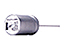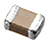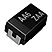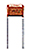• The cathode terminal material differs between the two. Standard TCs use manganese dioxide (MnO2) while conductive polymer types (i.e. ROHM's TCO/TCTO series) feature an organic substance, which is less combustible and provides much lower ESR (Equivalent Series Resistance) characteristics (typically 1/10th). This makes them ideal for high frequency and large current applications.

Conductive Polymer TC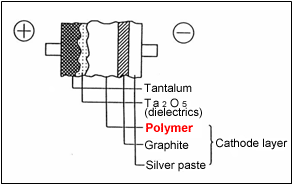Standard TC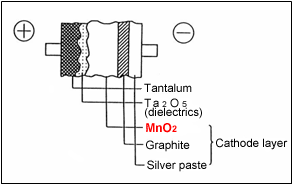• The allowable ripple voltage must take a sine wave shape (see below). For other waveform types, please contact a ROHM representative.
The permissible temperature increase due to ripple voltage/current is 5ºC max. At temperatures above this level the dielectric will begin to deteriorate, resulting in possible short-circuits.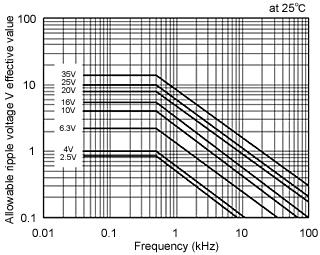In order to determine the limits for the allowable ripple voltage at high temperatures, please refer to the following formulas:
E=allowable ripple voltage
E Max.(at50ºC)=0.7×E Max.(at 25ºC)
E Max.(at85ºC)=0.5×E Max.(at 25ºC)
E Max.(at125ºC)=0.3×E Max.(at 25ºC)
• The allowable ripple current is calculated from the following formulas, which take into account ESR and power dissipation, which differ depending on the package type.
P=I2R
I = √(P/R)